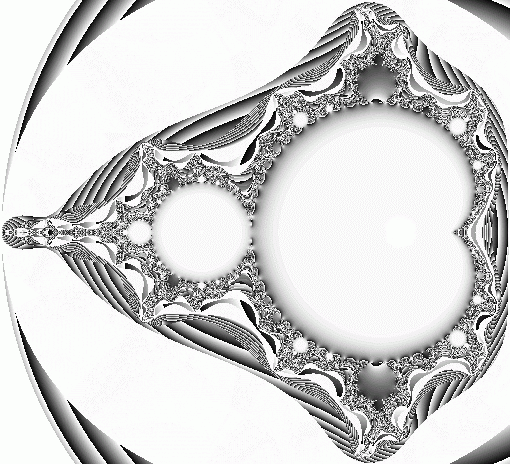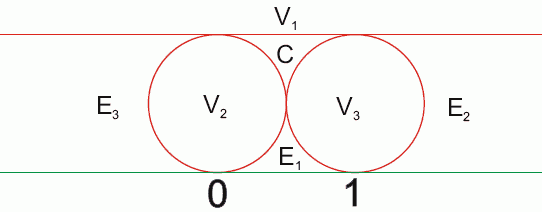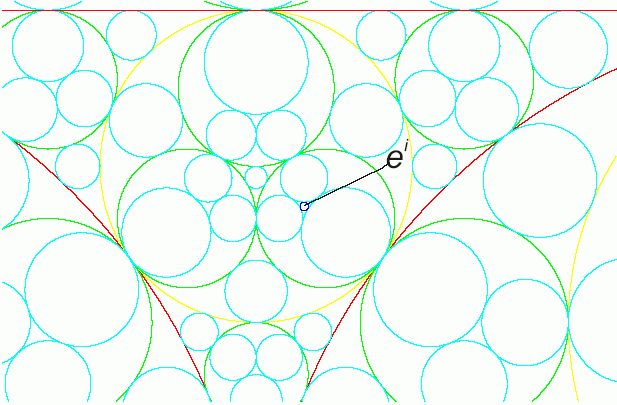## Gaussian Numbers

Ed Pegg Jr., March 15, 2004

I learned about the complex plane at about the same time I learned of the quadratic formula.  With that, I knew x^2 - 2 x + 17 had a solution in complex numbers.  I didn't come across complex numbers again until I saw the Mandelbrot set for the first time.Figure 1.  The Mandelbrot Set, colored by average differences between iterations.

After that, I was fascinated by the complex plane.  I remember a fellow enthusiast showing me the basic Mandelbrot set.  "I managed to get it to render in just 8 hours!"  Computers have sped up tremendously since then.  There are dozens of free programs for Mandelbrot set explorations, allowing real-time zooms into all manner of beautiful structures.  I can particularly recommend Fractal Extreme and Fractint.

Outside of fractals, one rarely sees complex numbers in the newspapers.  For example, the number 220996011 - 1 recently made headlines as the new largest prime number, as part of the Great Internet Mersenne Prime Search.  A lesser headline was made by the number 5359•25054502+1, found by the distributed attack on the Sierpinski Problem.  The Collatz problem has been tested out to 262•250 by the 3x+1 search.  Many other distributed problems are listed by Aspenleaf Concepts, Inc.

Similar searches for Gaussian Integers are unknown to me.  For complex numbers, the Zetagrid project has collected the first 687 billion zeroes of the Riemann Zeta function.  1239587702.54745 is an interesting discovery, but it isn't a Gaussian integer.  For almost every interesting number theory question, there is a similar question in the Gaussian integers.

For example, the Fermat-Catalan conjecture looks for high powers summing to other high powers.   Ten examples are known: 1p + 23 = 32 (p > 2), 25 + 72 = 34, 132 + 73 = 29, 27 + 173 = 712,  35 + 113 = 1222,  338 + 15490342 = 156133,  14143 + 22134592 = 657,  92623 + 153122832 = 1137,  177 + 762713 = 210639282,  and 438 + 962223 = 300429072.  In Gaussian integers, Fred W. Helenius and myself have found 7 examples: (8+5i)2 + (5+3i)3 = (1+2i)7, (20+9i)2 + (1+8i)3 = (1+i)15, (5i)^3 + (3i)^7 = (34-34i)^2,  (49+306i)^2 + (1+2i)^7 = (27+37i)^3, (44+83i)^2 + (31+39i)^3 = (5+2i)^7,  (19+36i)^2 + (1-i)^13 = (9+8i)^3, and (2+i)^4 + (1+i)^9 = (5+4i)^2.

The ABC conjecture looks for sums a+b=c where a, b, and c are all divisible by high powers.  For example 73 + 310 = 211•29.  This problem could be readily adapted to the Gaussian integers.

Beal's Conjecture is false for Gaussian integers.  Fred W. Helenius found a single counterexample: (-2+i)^3 + (-2-i)^3 = (1+i)^4.  Whether there are more counterexamples, I don't know.

A Sierpinski Number is a number k such that k•2n + 1 is always composite.  I discovered that 10+3i is a Sierpinski number.  On the other hand, for 26+i, you won't find a prime until (26+i)•24890 + 1.  I don't know of any distributed searches for large Gaussian primes.

The Catalan Conjecture has been solved for normal integers. Fred W. Helenius found several solutions among the Gaussians. (78+78i)^2 + (23i)^3 = i,  1 + (1-i)^5 = (1+2i)^2,  i + (11+11i)^2 = (3i)^5.

Continued Fractions can represent any real number.  For example, Pi can be represented as a continued fraction. Pi = {3, 7, 15, 1, 292, 1, 1, 1, 2, 1, 3 ...}.

Pi = 3.14159265... =Figure 2.  Pi as a continued fraction.

It turns out that complex numbers can also be represented as fractions.  The best method at the moment was devised by Asmus Schmidt. It works by careful consideration of 8 matrices.Figure 3.  The eight important matrices for complex continued fractions.

A complex number, for example ei, can be represented as a series of these matrices: c c v3 c c v3 v3 v3 c c v3 v3 v3 v3 v3 c c and so on, in this case.  If you multiply these matrices together,is the result.  If the top and bottom are added up, the fraction (1501 + 820 i)/(1501 - 820 i) can be made, which approximately equals 0.5403023380 + 0.8414709642 i.  ei starts off 0.5403023059 + 0.8414709848 i.  So how does this work?  It turns out that these matrices can be used to divide up the complex plane into 8 parts, in the first generation.  After that, each triangular region is divided into 4 parts, by adding a circle. That part works like an Apollonian packing. In addition, each circle is divided into 8 regions, by adding 3 tangent circles.Figure 4.  The first generation of Asmus Schmidt's complex continued fraction method.

In the third generation, we already have quite a few circles.  This represents all the regions representable by 3 of the matrices above.Figure 5.  The third generation of Asmus Schmidt's complex continued fraction method.

Here is a portion of Generation 4.  We are starting to zoom in on ei, positioned within the generations as c c v3 c c, so far.Figure 6.  The fourth generation of Asmus Schmidt's complex continued fraction method.

I wanted to look at a zoomed-in picture of ei at generation 50 or so, but I haven't quite figured it out how to draw all the circles. Specifically, I need to draw three tangent circles inside a larger circle, given 3 points of tangency.  I'll find it. Just as some things deserve more study, other things deserve a nice picture.

References:

ABC Conjecture page, http://www.math.unicaen.fr/%7Enitaj/abc.html.

The Beal Conjecture, http://www.math.unt.edu/%7Emauldin/beal.html.

Great Internet Mersenne Prime Search, http://www.mersenne.org/prime.htm.

Internet-based Distributed Computing Projects, http://www.aspenleaf.com/distributed/ap-math.html.

On the 3x+1 Problem, http://personal.computrain.nl/eric/wondrous/index.html.

Asmus Schmidt.  "Ergotic Theory of Complex Continued Fractions", Lecture Notes in Pure and Applied Mathematics, vol 147. p 215-276.  1993.

Seventeen or Bust, http://www.seventeenorbust.com/.

Eric W. Weisstein. "Apollonian Gasket", "Catalan Conjecture", "Collatz Problem", "Complex Plane", "Fermat-Catalan Conjecture", "Mandelbrot Set", "Quadratic Formula", "Sierpinski Number".   From MathWorld--A Wolfram Web Resource. http://mathworld.wolfram.com/

Zetagrid, http://www.zetagrid.net/.

Comments are welcome. Please send comments to Ed Pegg Jr. at ed@mathpuzzle.com.

Ed Pegg Jr. is the webmaster for mathpuzzle.com. He works at Wolfram Research, Inc. as the administrator of the Mathematica Information Center.